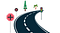# Mutually Exclusive, Independent and Dependent Events-Bayesian Inference

## Probability Topic- Bayesian Inference Tutorial-3

Before start explaining about these topics, it is advisable to read the previous topics on this tutorial series.

One thing I do before explaining this topic. Let’s see what google’s definition of this topic.

Okay, you can see the definition of google on the mutually exclusive events above. Come and see what does the first line says.

Two events are mutually exclusive if they cannot occur at the same time.

What are the events cannot occur at the same time? Let’s see a random four events about this,

1. A person cannot run forward and backward at the same time.
2. A person cannot stand and sit at the same time.
3. A person cannot laugh and cry at the same time.
4. A person cannot live in New York and California at the same time.

So as per the first line, we can say the above events are mutually exclusive events.

Now interpret the second line…

If two events are mutually exclusive, then the probability of either occurring is the sum of the probabilities of each occurring.

Isn’t? Let’s check whether it is true or not.

As we have already seen in the Intersection and Union of sets tutorial, the two mutually exclusive events do not intersect. This means two events haven’t common elements among them.

So the probability of either occurring should be the sum of the two events. In formula,

P(A ∪ B) = P(A)+P(B)

For example, consider tossing a fair coin. The probability of getting Heads and Tails is 0.5 each. We already know that the probability value always falls between is 0 to 1.

If someone comes and asks you the probability of falling either one of the two sides of a fair coin, how do you answer? Will he accept your answer if you merely say the answer to him. Let’s do the experiment in front of him and show him visually.

P(Heads ∪ Tails) = P(Heads) + P(Tails) = 0.5 + 0.5 = 1

So you can say the probability of getting heads or tails is 1.

Because no chances are there for anything else to happen. If the probability is 0, then we can surely say the event will not happen for sure. Probability value changes from 0 to 1 depends upon the possibility of an outcome.

It’s a pretty much easy concept to understand. What is the concept is, if the probability of the one event occurs does affect the other one, it is considered as the independent events.

Sounds like gibberish?

Let’s understand it with an example,

Consider two events occur in sequence,

1. Tossing a coin.
2. Picking a diamond card from a pack of cards.

The probability of getting heads from tossing a coin doesn’t affect the probability of getting a diamond card from a pack of cards.

The probability of both events occurring is 0.125

If you want to calculate the probability of both different events occurring, you can directly apply the above formula and find the possibility. You would understand the concept even better after grasping the concept of dependent events.

This is the straight opposite to the concept of Independent events. If the occurrence of one event influences the probability of another, the events are dependent. Without wasting much time on the definition, let’s go to the example.

Let’s imagine a bag having three red balls and three blue ones. The probability of picking the red ball in the first attempt without seeing it.

Event A

Probability of getting Red ball in the first attempt = 3/6 = 0.5

Oh, we have picked the red ball. (Imagine)

Okay, now let's do the same again without replacing the ball again into the back which we took in the first attempt. Now what is the probability of getting the red ball again?

Event B

Consider P(R) is the probability of getting red balls.

P(R) = 2/5 = 0.4

Compare the probability of event A and B

Now the probability of picking the red ball second time is affected by the first event. So this is dependent events.

If we do the same experiment with replacing the balls again into the bag. The probability would be the same for both the events. Then, it would be independent events.

Easy, Right?

After reading all this, it may be hard to find the difference between mutually exclusive and independent events. Both are completely different. I restate the definition of both again here.

Mutually Exclusive Events : Two events are mutually exclusive if they cannot occur at the same time.

Independent Events : If the occurrence of one event doesn’t influence the other, then the events are independent.

As a matter of fact, mutually exclusive events are dependent events.

Consider tossing a coin, the results are mutually exclusive. Because we cannot get the heads and tails in a single toss. At the same time, the occurrence of one preventing another one from happening. Hence it is definitely not an independent event.

Now consider tossing two coins, the results are independent and also not mutually exclusive for obvious reasons.

Cool!

As we are heading towards understanding the Bayes’ inference, now we have a great understanding of the basic things. Still some more topics we should learn to completely understand the Bayes’ theorem. We will see about those in subsequent topics.Conditional probability, Additive, and Multiplication rule of probability are on the way in our tutorial Series